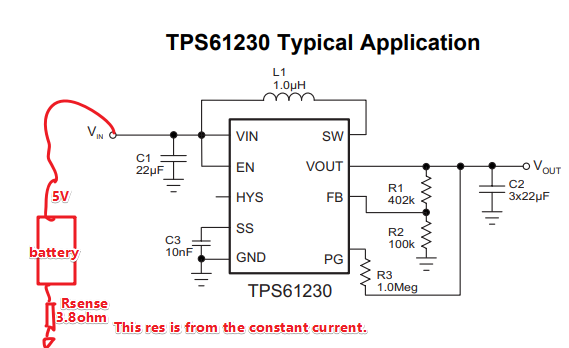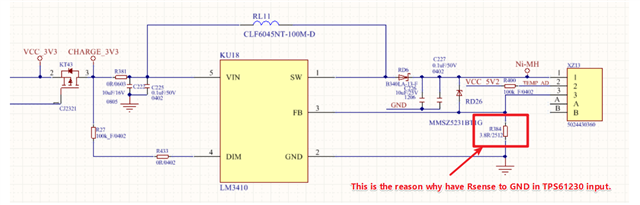If you have a related question, please click the "Ask a related question" button in the top right corner. The newly created question will be automatically linked to this question.

TPS61230: The input GND have res to GND

Part Number: TPS61230

Hi Team,

My customer now use TPS61230.

But the input from backup battery has a 3.8 ohm to GND.But with the 3.8 ohm from Front circuit, TPS61230 circuit will oscillate; When customer disconnected Front circuit, which means no 3.8ohm.TPS61230 could work normally.

May I ask how could TPS61230 could work normally even with 3.8 ohm to GND?• Hi Amelie,

what is the output voltage/ current in your application? the 3.8R will limit the current out of the battery. if the output power is high, the input voltage for the boost will drop and trigger UVLO.

• Hi Jasper,

The input is 4V

The output is 5.1V/500mA..

I am not sure whether I understand your description.

If input=4V, because of Rsense=3.8ohm, so the input current is 4V/3.8ohm=1A? Because the output power is 5.1V*500mA. So the input voltage is 5.1V*500mA/1A=2.5V? So it will trigger UVLO?

But why the input current is depend on the demand?

• Hi Amelie,

the power into the tps61230 must be higher than TPS61230's output power.

if there is a  3.8R in series with the battery, the maximum power out of the is battery only 1W. this is lower than the output power 5*500mA=2.5W.

So the circuit didn't work.

• Hi Jasper,

How to calculate the maximum power out of the battery? Why it is 1W?

• Hi Amelie,

The power out of the battery is (VBAT-IBAT*3.8)*IBAT. the IBAT is the current out of the battery. then we can find the maximum value of  (VBAT-IBAT*3.8)*IBAT

• Very clear and thanks！